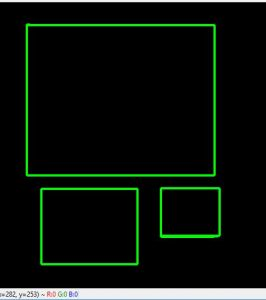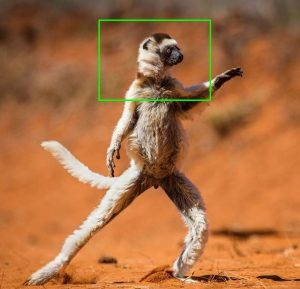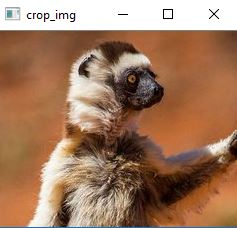GeeksforGeeks App
Open AppBrowser
Continue

# Draw a rectangular shape and extract objects using Python’s OpenCV

OpenCV is an open-source computer vision and machine learning software library. Various image processing operations such as manipulating images and applying tons of filters can be done with the help of it. It is broadly used in Object detection, Face Detection, and other Image processing tasks.

Let’s see how to draw rectangular shape on image and extract the objects using OpenCV.

 `# Python program to extract rectangular``# Shape using OpenCV in Python3``import` `cv2``import` `numpy as np`` ` `drawing ``=` `False`     `# true if mouse is pressed``mode ``=` `True`         `# if True, draw rectangle.``ix, iy ``=` `-``1``, ``-``1`` ` `# mouse callback function``def` `draw_circle(event, x, y, flags, param):``    ``global` `ix, iy, drawing, mode``     ` `    ``if` `event ``=``=` `cv2.EVENT_LBUTTONDOWN:``        ``drawing ``=` `True``        ``ix, iy ``=` `x, y``     ` `    ``elif` `event ``=``=` `cv2.EVENT_MOUSEMOVE:``        ``if` `drawing ``=``=` `True``:``            ``if` `mode ``=``=` `True``:``                ``cv2.rectangle(img, (ix, iy), (x, y), (``0``, ``255``, ``0``), ``3``)``                ``a ``=` `x``                ``b ``=` `y``                ``if` `a !``=` `x | b !``=` `y:``                    ``cv2.rectangle(img, (ix, iy), (x, y), (``0``, ``0``, ``0``), ``-``1``)``            ``else``:``                ``cv2.circle(img, (x, y), ``5``, (``0``, ``0``, ``255``), ``-``1``)``     ` `    ``elif` `event ``=``=` `cv2.EVENT_LBUTTONUP:``        ``drawing ``=` `False``        ``if` `mode ``=``=` `True``:``            ``cv2.rectangle(img, (ix, iy), (x, y), (``0``, ``255``, ``0``), ``2``)``     ` `        ``else``:``            ``cv2.circle(img, (x, y), ``5``, (``0``, ``0``, ``255``), ``-``1``)``     ` `img ``=` `np.zeros((``512``, ``512``, ``3``), np.uint8)``cv2.namedWindow(``'image'``)``cv2.setMouseCallback(``'image'``, draw_circle)`` ` `while``(``1``):``    ``cv2.imshow(``'image'``, img)``    ``k ``=` `cv2.waitKey(``1``) & ``0xFF``    ``if` `k ``=``=` `ord``(``'m'``):``        ``mode ``=` `not` `mode``    ``elif` `k ``=``=` `27``:``        ``break`` ` `cv2.destroyAllWindows() `

Output:Above piece of code will work with only black background image. But rectangles can be drawn to any images. We can write a program which allows us to select desired portion in an image and extract that selected portion as well. The task includes following things –

• draw shape on any image
• re-select the extract portion for in case bad selection
• extract particular object from the image

 `# Write Python code here``# import the necessary packages``import` `cv2``import` `argparse`` ` `# now let's initialize the list of reference point``ref_point ``=` `[]``crop ``=` `False`` ` `def` `shape_selection(event, x, y, flags, param):``    ``# grab references to the global variables``    ``global` `ref_point, crop`` ` `    ``# if the left mouse button was clicked, record the starting``    ``# (x, y) coordinates and indicate that cropping is being performed``    ``if` `event ``=``=` `cv2.EVENT_LBUTTONDOWN:``        ``ref_point ``=` `[(x, y)]`` ` `    ``# check to see if the left mouse button was released``    ``elif` `event ``=``=` `cv2.EVENT_LBUTTONUP:``        ``# record the ending (x, y) coordinates and indicate that``        ``# the cropping operation is finished``        ``ref_point.append((x, y))`` ` `        ``# draw a rectangle around the region of interest``        ``cv2.rectangle(image, ref_point[``0``], ref_point[``1``], (``0``, ``255``, ``0``), ``2``)``        ``cv2.imshow(``"image"``, image)`` ` ` ` `# construct the argument parser and parse the arguments``ap ``=` `argparse.ArgumentParser()``ap.add_argument(``"-i"``, ``"--image"``, required ``=` `True``, ``help` `=``"Path to the image"``)``args ``=` `vars``(ap.parse_args())`` ` `# load the image, clone it, and setup the mouse callback function``image ``=` `cv2.imread(args[``"image"``])``clone ``=` `image.copy()``cv2.namedWindow(``"image"``)``cv2.setMouseCallback(``"image"``, shape_selection)`` ` ` ` `# keep looping until the 'q' key is pressed``while` `True``:``    ``# display the image and wait for a keypress``    ``cv2.imshow(``"image"``, image)``    ``key ``=` `cv2.waitKey(``1``) & ``0xFF`` ` `    ``# press 'r' to reset the window``    ``if` `key ``=``=` `ord``(``"r"``):``        ``image ``=` `clone.copy()`` ` `    ``# if the 'c' key is pressed, break from the loop``    ``elif` `key ``=``=` `ord``(``"c"``):``        ``break`` ` `if` `len``(ref_point) ``=``=` `2``:``    ``crop_img ``=` `clone[ref_point[``0``][``1``]:ref_point[``1``][``1``], ref_point[``0``][``0``]:``                                                           ``ref_point[``1``][``0``]]``    ``cv2.imshow(``"crop_img"``, crop_img)``    ``cv2.waitKey(``0``)`` ` `# close all open windows``cv2.destroyAllWindows() `

Run : Save the file as capture_events.py and for testing select a demo picture which is located in the same directory. Now, execute the following command –

`python capture_events.py --image demo.jpg`

Output: First select the desired portion from the image. In addition, we can remove bad selection by pressing ‘r’ as programmed for making a new proper selection.Fig: Selected Portion

Now after selecting a proper selection like above, just press ‘c’ to extract, as programmed.
Fig: Cut PortionMy Personal Notes arrow_drop_up